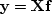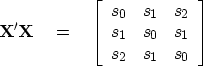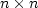Next: Setting up any weighted Up: WEIGHTED ERROR FILTERS Previous: Gain before or after

## Meet the Toeplitz matrix

The solution to any least-squares problem proceeds explicitly or implicitly by finding the inverse to a covariance matrix. Recall the basic filtering equation (),(20)
which we can abbreviate by.To gain some understanding of your cultural heritage in time-series analysis, form the covariance matrix,(21)
where the elements st are lags of the autocorrelation of xt. This covariance matrix is an example of a Toeplitz matrix.  When an application is formulated in the frequency domain, you may encounter a spectrum as a divisor. When the same application is formulated in the time domain, you will see an autocorrelation matrix that needs inversion.

The Toeplitz matrix is highly structured. Whereas anmatrix could contain n2 different elements, the Toeplitz matrix contains only n elements that are different from each other. When computers had limited memory, this memory savings was important. Also, there are techniques for solving least-squares problems with Toeplitz covariance matrices that are much faster than those for solving problems with arbitrary matrices. The effort for arbitrary matrices is proportional to n3, whereas for Toeplitz matrices it is n2. These advantages of Toeplitz matrices were once overwhelming, although now they are rarely significant. But because old methods linger on, we need to decide if they are warranted. Recall that we wrote three convolution programs, contran(), contrunc(), and convin(). You can verify that a Toeplitz matrix arises only in the first of these. The other two represent different ways of handling boundaries. Letbe a diagonal matrix of weighting functions. You can also verify that the covariance matrixis not Toeplitz. Thus, Toeplitz matrices only arise with uniform weighting and transient boundary conditions. If the only tool you have is a hammer, then everything you see starts to look like a nail. In earlier days, and by inertia even today, convolution applications tend to be formulated as uniformly weighted with transient boundaries. This is a pitfall.

 Toeplitz matrices are associated with elegant mathematics and rapid numerical solutions. Applications that are solvable by standard methods have historically been cast in Toeplitz form by imposing simplifying assumptions. This is risky.

The approximation (19) becomes reasonable when the weights are slowly variable, i.e., when wt is a slowly variable function of t. In practice, I think the approximation is often justified for slow t2 gain but questionable for automatic gains that are faster. Compared to Toeplitz methods of solving equation (19), the CG method of solving (18) is slower. Here we are going to see how to solve the problem correctly. If you want to solve the correct problem rapidly, perhaps you can do so by solving the approximate problem first by a quasi-analytic method and then doing a few steps of CG.Next: Setting up any weighted Up: WEIGHTED ERROR FILTERS Previous: Gain before or after
Stanford Exploration Project
10/21/1998